MOUND CONFIGURATIONS ON THE
MARTIAN CYDONIA PLAIN

Horace W. Crater
The University of Tennessee Space Institute

and

Stanley V. McDaniel
Sonoma State University

Abstract

We show that a group of mound-like formations in the Cydonia area of Mars of relatively small and nearly uniform size have relative positions that  repeatedly display  symmetries  in the apparent form of related right and isosceles triangles. We also show that  these pairs cluster sharply in density about a certain value of the defining angle of those related  triangles and that on average the vertices of the triangles lie significantly closer to the measured centers of the mounds than those for fictitious mounds from a computer simulation.  Our computer simulation of the surrounding features and the mound  formations themselves demonstrates that the numerous examples of  these symmetries,  the resultant clustering about certain proportions, and the relative precision of the vertices to the mound centers are not compatible with random geological forces.  We have thus uncovered an anomaly of number, geometry and precision.  In order to give a quantitative measure of this anomalous distribution of mounds we determine the likelihood that we will make an error by rejecting the null hypothesis. This level of significance we find for our test is p~15.5 x 10-6 .  That is, in a million trials, the repetition of the frequency of appearance of these triangles,  greater than or equal to the observed  (19) in the actual data, and with the observed or greater precision, is about 152.5.  In this computer simulation the average number of appearances is about 6, with a standard deviation of about 2.  In 95% of the computer simulations, the distance of the vertices of the triangles was, on average, further from the (fictitious) mound centers than for the case of the actual mounds

The authors wish to acknowledge helpful input on geological matters from Erol  Torun, James Erjavec, Dr. Arthur Reesman and Dr. Robert Schoch, on issues of statistical techniques from  Dr. Richard Larson, Dr. Conley Powell, Dr. Ken Wheaton, and Gerry Zeitlin, and  on matters of geometry from Erol Torun and Dr. James F. Strange.  They also appreciated vigorous debate and useful exchanges from the above as well as Dr. Tom Van Flandern, Dr. Marie-Louise Kagan, Dr. Michael Zimmerman, Dr. David Webb, Dr. Halton Arp, Dr. Douglas Hal, Dr. Daniel Atkinson, James Oberg, John Legon, Cesar Sirvent,  Dr. Dave Rothery, and Dr. Ralph Greenberg. We are especially indebted to Dr. Mark Carlotto, Erol Torun, Daniel Drasin, Ananda Sirisena, Lan Fleming, Larry Reynolds, Newton Wright, and Linda Engels for supplying important electronic images, for technical assistance regarding their interpretation, and for general graphics support.

Introduction

During the 1976 Viking missions to Mars, several images (primarily NASA Viking frames 35A72, 70A11, 70A13, 561A25), taken over the area known as the Cydonia Plain, revealed some unusual surface formations. Over the intervening two decades various independent researchers have studied these formations. It has been their consensus that the area exhibits a degree of anomaly sufficient to warrant active investigation of the site by future space probes1.

Work by these researchers has focused primarily on several larger objects in the area (averaging about 1 square kilometer in size). In 1994 Horace W. Crater undertook to investigate the visually apparent geometric relationships found within a group of much smaller, hill-like features (here called for convenience mounds) located in the same vicinity (Crater 1994, 1995,1998,1999). The area where the mounds are located is near the controversial "Face" formation. However our analysis of the apparently anomalous character of the mound distribution is independent of questions regarding the formation of the "Face." Although exact knowledge of the morphology of these objects must await higher resolution images, the Viking images are of sufficient resolution to make feasible a quantitative study of their spatial relationships, from which certain reasonably secure conclusions may be drawn.

Subject Matter and Hypothesis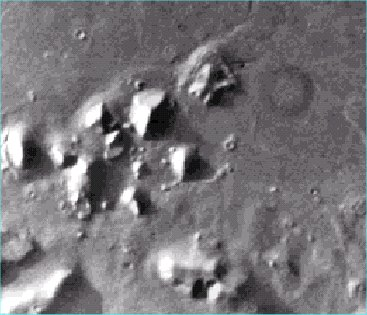Figure 1. A Portion of Viking Frame 35A72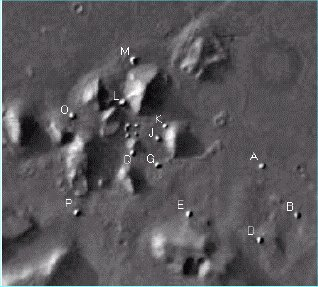Figure 2.  Letter designations for 12 mounds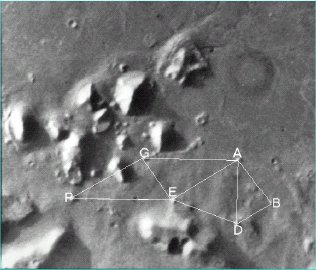Figure 3.  Visually evident parallel lines,  right and isosceles triangles

By the small mounds is meant a group of objects 0.1 to 0.2 km2 in size located in the region of Cydonia under study. Approximately sixteen objects in the area fall into this category. Figures 1 & 2 are for descriptive purposes and should not be used for measurement. In these small images some highlights may be mistaken for "mounds" but are actually bright spots on larger features.

In Figure 2 the mounds are brightly enhanced relative to the background and letter designations are shown for twelve of the sixteen, excepting only four that are tightly clustered in the left center area of the image, where the letters would be too crowded. These four are discussed later on (in Section V A).

These objects are not tightly clustered but are separated in some cases by as much as three km. They are clearly distinguished from other land forms in the area not only by their size in relation to the larger surrounding features whose geological nature is evident (craters, mesas, and mountain-like features) but also by their brightness, which causes them to stand out from the general background. Although the 47-meter/pixel resolution is insufficient to reveal their fine structure, in the best image enhancements some of the mounds appear to cast a distinct shadow that comes to a point.

They may be what geologists call sand boils, pugs or perhaps dreikanters (three-sided pyramidal shapes resulting from wind erosion). Their exact intrinsic nature is not immediately relevant to this discussion.

There are several objects in Figure 1 (other than the ones lettered in Figure 2) that are either a) significantly larger, b) parts of larger structures obscured by shadows, c) not clearly distinguished from other structures they appear attached to, d) have significantly reduced albedo relative to other mound-sized forms, e) associated with small pedestal craters

Attention was first drawn to these objects because of the apparent regularity of arrangement among the six that lie in the relatively open area south of the larger formations, comprising mounds P, G, E, A, D, and B (see Figure 3). Specifically, within the margin of error for measurement and visually obvious, (1) triangle EAD is an isosceles triangle; (2) lines drawn from the estimated centers of mounds PG, EA, and DB are parallel; (3) lines drawn for mounds PE and GA are also parallel, forming a parallelogram PGEA; (4) and triangles drawn for mounds PGE and GEA are right triangles containing the same angles and of the same size. Not as obvious visually but clear enough upon measurement, (5) triangles drawn for mounds GAD, and ABD are again right triangles containing the same angles as PGE and GEA although different in size while the right triangle EAB has the same angles and same size; and (6) the isosceles triangle EAD  has angles such that if it were bisected at the vertex at mound D, then it would be split into two right triangles that closely match the right  triangle ADB, in both angles and size. These visually detectable relationships are shown in Figure 3 (detailed discussion of measurements in order of discovery to follow later). The visible regularities, and the relative isolation of the six mounds involved, warrant a more detailed investigation of the geometric characteristics of the mound configuration.

Methodological Considerations

Investigation of the geometric relationships between these mounds takes the form of a test of what may be called the random geology  hypothesis. This hypothesis presupposes that the distribution of the mounds in the specified vicinity (i.e. the area of other recognized anomalous formations), however orderly it may seem, is consistent with the action of random geological forces. Our question is: Does the random geology hypothesis succeed or fail in the case of the small mound configuration at Cydonia?

The random placement of a number of mounds may result in any number of orderly arrangements, seemingly unlikely to occur by chance. The probability, though small, that any one such arrangement is a result of random forces is not very meaningful. The reason is that there may be many equally orderly arrangements that also may seem intuitively unlikely. For example, in the case of  an arrangement of three mounds in the form of an isolated isosceles triangle, the probability of that particular isosceles  triangles occurring by chance is not as meaningful as is  the probability of any isosceles triangles occurring by chance.

On the other hand, if a relatively simple pattern, precise within reasonable limits, occurs with unusual redundancy, then it becomes meaningful to focus a priori on that particular pattern. In our visual inspection of six mounds (GABDEP), we found evidence of an initial redundancy in the form of a series of similar right triangles each of which appears to be related to the isosceles triangle ADE (as described above, bisecting the isosceles produces two equal right triangles geometrically similar to the right triangle outlined by the mounds in the figure). This observed redundancy of associated right and isosceles triangles may well be a result of random placement. To test whether this is the case , we determine by computer simulation the odds that the number of appearances of these triangles will be greater than or equal to that observed. Due to the finite size of the mounds and the many triangles that can be drawn between vertices chosen from a multi-mound configuration, numerous appearances could very well be compatible with a random distribution. Under  the random geology hypothesis we would expect odds on the order of 1 in 100 or greater.  This is a common value for the level of significance used in statistical tests.  It meaning is that we would reject the null hypothesis (our random geology hypothesis) if those odds were less than 1 in 100.  The level of significance we find in this paper is (15.52.5)x 10-6 .   Technically this gives us the fraction of the time we would commit an error of the first kind. This is defined a the error one makes by rejecting the null hypothesis when in fact it is true (Brownlee, 1965).

In Section I we present a detailed description of our criteria for selection of the mounds and tabulate their positions and sizes.  For comparison we also give the size range of the larger features near the mounds. In Section II we describe  the primary features of their apparently symmetrical arrangement, namely repeated appearances of similar isosceles and right triangles. We discuss the nature of the relations discovered and describe our methodology for testing the random geology hypothesis in Section III, including a  display of  the results in graphical form.  In Section IV we determine the level of significance of our test and in Section V we describe further tests.

1.  Selection Criteria for Mound-Like Land Forms

To those unfamiliar, or only casually familiar, with the Viking images, it may appear as though our selection of the mounds is arbitrary among a field of many possibilities. The reference images accompanying this paper do not show the detail that high quality orthorectified enhancements display. (Orthorectification corrects for the distortion of angular placements of surface features in the image due to a non-overhead camera viewing angle.) Intimate familiarity with research quality images, however, reveals quite a different picture. There is a fairly obvious, clear-cut distinction between the mounds and the much larger structures in the area. In this section we present our selection criteria for the formations we have designated with the generic term mound.

Measurements given below are obtained from orthorectified digital images processed from the original Viking data by Dr. Mark J. Carlotto and Mr. Erol Torun, both highly qualified professionals in image processing and cartography respectively. Serious research requires reference to these enhancements or enhancements of equal quality. (The authors would welcome replication of their work and will provide orthographically rectified, research-quality images upon request.)

A. Size and Uniformity. The size of the mounds is noticeably smaller than the large structures in the area. The mounds are more or less uniform in size and general shape, with an average area of 76 square pixels, or about 0.167 square kilometers. In contrast the larger features in the area are on the order of 1 square kilometer or greater. The estimated areas (in km2) of the 12 lettered mounds are given in Table I.  We estimated the areas by counting the number of times the X and Y counters on our imaging software changed values as we guided the pointer from one extreme side to the other.  Using 47 meters per pixel we obtain the areas listed in the table. The uncertainties are on the order of 0.01 km2  For comparison, the average area of the larger objects is about 1438 square pixels (about 3.16  km2), with the largest being 4425 square pixels and only three being less than 700 square pixels (at 490, 400, and 225 square pixels). The smallest of the larger objects is nearly double the largest mound size.  We also list in the table the relative location of all the mounds relative to mound G.  Mound G itself is located at approximately 40.9 degrees north latitude and 9.8 degrees west longitude.

TABLE 1 Areas and Relative Positions of Mounds

 Mound Area in km2 N/S from G in km E/W from G in km G 0.18 0 0 A 0.13 -0.05 4.8 D 0.14 -3.5 4.7 E 0.15 -2.3 1.5 B 0.12 2.3 6.4 P 0.18 -2.2 -3.7 K 0.16 1.8 4.2 J 0.15 1.2 4.7 L 0.22 2.9 -1.5 M 0.29 4.9 -0.9 Q 0.11 0.6 -1.1 O 0.22 2.3 -3.8

B. Albedo. Unlike some of the small hill-like features of similar size (for example the three or four formations in the area bound by mounds A, B, D and the two formations between E and P), the mounds we choose  appear to have a high albedo. It is the relatively uniform brightness and similar size of the mounds that causes them to stand out noticeably against the background. On average the mounds are about 90% as bright as the brightest areas on the larger formations, and about 125% as bright as the mean background brightness. The sun angle in Fig. 1 (Viking frame 35A72) is about 10 degrees. In the other Viking frame (70A11) the angle is about 27 degrees. In both cases the surfaces of those mounds on the sun-facing side have this albedo display.

C. Shape. In most cases the shadows of these formations taper to a point. This latter feature is especially noticeable in mounds A, B, D, E, P, L, Q.  On mounds G and A, in the best enhancements, there are clearly visible surfaces indicating a faceted structure.  Most other mounds display some evidence of triangular like sides. Although  mound O looks to be more rounded and does not appear to have such sharp features, a further examination of  it from Viking frame 70A11 reveals angular surface features like the other mounds. We emphasize, however, that the shape is not the focus of this study.

D. Isolation. There are a  number of features with significant albedo which at first sight look like mounds, but on closer inspection are found to be projections on larger structures. For example, this is true for four or five items found just above mound P. Three of these directly above mound P in Figure 2 are actually protrusions on a much larger formation. The rightmost of the five (at about 2:00 from P) looks like an isolated mound in Figure 1 (Viking frame 35A72) but is seen in frame 70A11 to be the illuminated peak of a protrusion extending from that larger formation. The leftmost (at about 11:00 from P) is eliminated because it has significantly reduced albedo relative to the other mound-size forms (it is not sun blocked).The mound-like structure to the far left center of the image is also a protrusion on a larger formation. In contrast to these, mound Q is very near a larger formation but is clearly separated from it. The same can be said for mounds L and M.

There is a close doublet formation about two thirds of the way up on the left portion of the figure, out on the open plain. We leave this out because it lacks albedo and its doublet structure makes its position ambiguous. We also leave out the twin mound-like forms that terminate a chain-like feature extending out to the east of the large oval structure adjacent to mounds K and J. These are clearly part of the chain and have a significantly lower albedo. Similarly a few low, rounded hill-like features near mound B do not have the same sharpness or albedo as B and so they are eliminated. We also eliminate from our candidates structures that appear to have clearly defined causative factors such as obvious association with a pedestal crater (there are several  such cases).

Attention has frequently been drawn to the four apparent mounds located roughly in the center of the larger structures and just to the left of mounds J and K. These four objects are arranged in a fairly regular pattern forming a cross, with an indication of a fifth, smaller mound-like feature  at the center of the cross. (Actually the northernmost of these objects does not strictly qualify as a mound by our definition, as on closer inspection it appears to be elongated, generally rectangular, and in the shape of an open L.) We do not include these four objects separately because the distances between them is on the order of their sizes, rendering angular measurements between them meaningless. We will, however, take them into account as a group later on in this study.

2. Geometric Measurements

It was noticed early on that mounds EAD appear to form an isosceles triangle.  This isosceles can be clearly discerned in Figure 3. The obvious visual symmetry is rather striking. To confirm this early impression, Crater made careful measurements. Since the mounds, though relatively quite small, are not geometric points, their angular relationships and the distances between them were measured initially from their centers estimated within a determined margin of error.
First the X and Y coordinates of the approximate centers of the mounds and their uncertainties were determined using an orthorectified image (one that takes into account camera angle). In the electronic imaging software (Photofinish), those coordinates appear as a pair of integer digits that change in whole  units as the mouse pointer is moved over the image. Each unit is referred to as  a pixel. X and Y coordinates were also obtained by direct measurement on hard copy. Three different orthorectified images based on two different Viking frames (35A72 and  70A11) were used to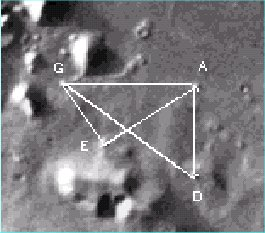Figure 4. Two similar Right Triangles.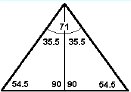Figure 5. The isosceles  and right triangles appear to be related.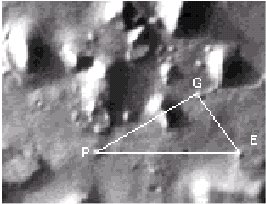Figure 7. Right triangle PGE, equal to AEG.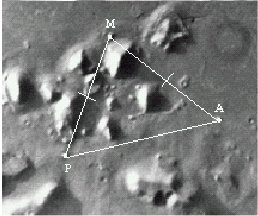Figure 8. Isosceles PMA, similar to ADE.
cross-confirm measurements. (The recent images taken by the Mars Global Surveyor displayed only a very few of the mounds and were not used in our analysis). The angle measurements presented here are from 35A72. Although the mounds are neither perfect circles nor squares, it is possible to locate their (approximate) geometric centers. The X and Y coordinates were recorded when the pointer was nearest this location. The uncertainty was normally one or two pixels either way based on numerous re-measurements. The mound sizes in the same units was 3-6 in either direction.

Then by means of the Pythagorean theorem and the law of cosines triangle EAD, as measured from those initial reference points, was found to contain the following angles: 71.1 3.2,  55.6 2.9,  53.2 2.7. Averaging the last two figures, this measurement is very close to an isosceles triangle of 71, 54.5, 54.5 degrees, which would fall well within the measurement error for the mound centers.

It had also been noticed by earlier investigators2  that the triangle formed by mounds EAG is a visually obvious right triangle. The base of the isosceles forms one side of this triangle (Figure 4.). Measurements from the apparent mound centers yield 88.7 3.9, 35.0 1.9,  56.3 2.8. Furthermore there is another visually apparent right triangle, with vertices GAD.  Its angles work out to be 88.2 2.70, 36.6 1.7, 55.2  2.4.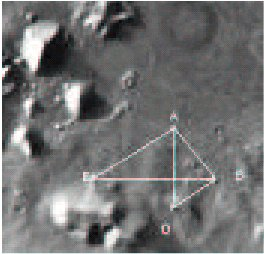Figure 6. Two more similar right triangles.
There are two remarkable features about these two right triangles. The first is that within the accuracy of the angular measurement, which averages about two and one-half degrees, the two right triangles (GAD, EAG) appear to have the same angles. The second is that these two apparently similar right triangles have angles that are consistent with one that bisects the isosceles triangle shown in Figure 3. Such a bisection would produce right triangles with angles of approximately 90, 35.5 and 54.5 degrees, consistent with the above right triangles (Figure 5).

A fifth mound (B) appears to form two other right triangles ABD and EAB (Figure 6 ). We find that triangles ABD and EAB contain respective angular measurements of 90.9 5.4, 52.6 3.3, 36.5 2.2 and 90.0 3.9, 55.2 2.4, 34.8 1.5. Within the measurement uncertainties they are both clearly similar to the two right triangles that appear in Figure 4.  There appears now to be a trend in the data, suggesting a quasipredictable phenomenon.  If so then this trend should continue within the other mounds.

A sixth mound P to the west of the five mounds is a  relatively isolated mound, yet we find that the triangle formed by mound P with mounds E and G (Figure 7) is again a candidate for a  right triangle (angles 92.1 3.8, 32.1 1.8 ,55.8 2.7) of approximately the same angles as appears in the four right triangles that appear in Figures 4 and 6. Furthermore, it is back to back and equal in size, within the margin of error, to two of those right triangles GEA and EAB (see Figs. 3,4  and 6).

Does this trend of redundancy include the isosceles as well as right triangles?

Including a  seventh mound M (Figure 8) adds another larger version (PMA) of the isosceles triangle ADE of Figure 3 with angles of 55.1, 54.7, and 70.3 degrees with similar uncertainties as before. Note that this triangle shares vertex A in common with the other isosceles ADE.

By empirical determination, following the clue provided by the visually obvious regularities, we have found an evident redundancy in the appearance of specifically related isosceles and right triangles within a calculated margin of error. As we shall discuss in Sec. III.C this redundancy appears to extend to the mounds to the north of the initial six mounds displayed in Figure 3. Is the mound distribution, despite these redundancies, consistent with the random geology hypothesis?

3   Statistical Analysis of the Mound Geometries

A legitimate criticism is that we have not accounted for the role of numerous other combinations among the mounds. (There are 220 triangles altogether defined by the 12 mounds). Nor has the above exploratory analysis taken into account other classes of right and isosceles triangles. We meet these objections by examining the set of all right and associated isosceles triangles, not just the ones encountered above.  Furthermore, in order to account for the above mentioned combinations we will devise a statistical test of the random geology hypothesis based on computer simulations.  In this section  these examinations and tests show decisively that these pairs of related right and isosceles triangles cluster sharply about a certain defining angle and that this peak is high above random expectations.

A  Mound Placement Analysis

The triangles found in our exploratory analysis above have a connection which we may quantify by defining their angles with one parameter which we will call  t.  In terms of this parameter the angles of an arbitrary right triangle can be written as simple linear functions of t and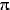with angles (in radians) defined by/2, (/4 + t/2), (/4 - t/2). (In degrees, 90, 45+ t/2, 45- t/2.)  The partnered isosceles would then have angles defined by (/2 - t), (/4 + t/2), (/4 +t/2). In degrees, (90- t), (45 + t/2), (45 + t/2). We then perform our analysis according to the following prescription:

A.1) We allow t to vary from 0 to 90 in one-half degree steps. Thus, defining t = (n-1)/2 we start with n = 1, in which case t = 0 . In this initial case the isosceles and right triangles are equivalent (both are 45 right triangles, i.e, 90 , 45 , 45 ).

A.2) At each step, using a computer program, we determine the angles in all possible triangles (220 altogether) that can be drawn between vertices located at the centers of each of the 12 mounds. From these, the program then identifies candidate right and isosceles triangles, ones with angles within a reasonable  prescribed limit of the ideal right and isosceles triangles described above for a given value of  t.  Once they are identified, we then vary the X and Y coordinates of the common vertices of the candidate triangles away from the center but within the areas of the mounds to see if a fit to the actual ideals can be achieved within a preset precision, (done here for two values, 5 degrees and 0.2 degrees). This variation is continued until one  achieves a  coordinated fit to the ideal triangles, with all of the angles agreeing within less than the preset precision level.  This is accomplished using a least square fitting routine. As this variation proceeds, some candidates drop out while on occasion some originally not identified may appear. The process continues until the maximum possible number of right triangles and associated isosceles triangles (with angles defined as above in terms of t) have been fit within the precision level indicated.

A coordinated fit requires that the same vertex within any given mound is used for all the triangles having one vertex sharing that mound, not shifted about arbitrarily within each mound to accommodate each triangle separately. As an example of what this means we refer to Figure 3 which represents a six mound coordinated fit involving similar right triangles and related isosceles. This stringent requirement for a coordinated fit severely restricts the number of chance matches. Note that the mound areas and placements could very well be such that the ideal for a given t would be impossible to fit, or very few triangles would achieve a coordinated fit.

Once this procedure (A.1-A.2) is finished we record the number (N(t)) of those types of triangles achieving a coordinated fit for the given value of t. (For example, for t = 10 the number of triangles achieving a coordinated fit within a 5 degree precision level is 15 triangles, or (N(t)) = 15.)

A.3)  The above two steps  are repeated for n = 2, ..., 181 corresponding to t = 0.5 to t = 90 with N(t) recorded at each step.

A.4) The above analysis, (A.1)-(A.3), is repeated for both levels of precision (5 degrees and 0.2 degrees).  The results are then plotted together with expected random distributions, as described below (see Figs. 9 and 10).

B  Simulated Mound Placement Analysis

In order to test for the validity of the random geology hypothesis we make a computer simulation of randomly distributed fictitious mound locations. Since the designated region contains not only mounds, but ten much larger structures, we  design a simulation containing both types of features, as follows:

(B.1.)  First 10 large circular regions are distributed randomly by computer, using a random number generator,  over an area comparable to the area in which the Cydonia mounds appear (about 67,000 square pixels) in such a way that they do not overlap.  The areas of the fictitious larger features are chosen randomly to lie within the range of areas of the actual larger features. The individual areas of the ten larger structures are  tabulated as follows (from greatest to least, in square pixels): 4425,  2350, 2040, 1950, 1040, 740, 720, 490, 400, and 225.

(B.2)  Next,  a  set  of 12 fictitious mound placements is generated also  using a random number generator. Each set of fictitious mound center locations is thus randomly scattered over the chosen area such that, like the actual data, the mounds do not overlap with the larger structures nor with each other. In both cases (B.1) and (B.2) the randomized data is constructed as follows

(a) First the random number generator provides two random numbers between 0 and 1. These are used to fix the X and Y coordinates, within an area one unit square, for the center of one of the fictitious mounds or large structures. This is repeated for each fictitious mound and larger structure.

(b) Second, this unit area and the center coordinates of the set of fictitious mounds and large structures are scaled so that the unit area becomes equal to the number of pixels of the general area in which the actual mounds are located (again, in the image this about 67,000 square pixels, or about 148 km2).

(c) The areas of the fictitious mounds, also in square pixels, are chosen randomly by computer from the  range of areas of the actual Cydonia mounds just as the areas of the fictitious larger structures are constructed randomly from the range of areas of the  the actual large structures.

(B.3).  Steps (A.1) and (A.2) that were taken for the actual mounds in the previous subsection are repeated here for the 12 fictitious mounds. A hit is recorded if and only if the vertices of the special right and related isosceles  triangles contained in the model lie within the confines of the fictitious mound areas of a given fictitious mound configuration and the angles are within the preset limit of precision.  The maximum number of hits is obtained by the same coordinated fit procedure (see 1b) used for the real mounds. Call this number Ni(t), in which i labels the run number.

Note that this number Ni(t) is sensitive to the size of the general area chosen to enclose our randomly generated mounds and large structures. As mentioned above, that area is chosen based on the actual image (see Figure 1).  There is an a priori reason for concentrating on this region (as opposed to ones far outside the boundary). This is the primary region that includes the features that have been cited by other researchers as indicating possible anomalous structures. In other words we did not randomly select this area for study.

The area which includes those other  structures is significantly greater than the portion of the image we have presented. However, this greater area has, relatively speaking, very few other mounds. If the area chosen both for our real data and the simulated data was taken to be that large, then since the number of real mounds would not be substantially increased, on average the fictitious mound centers would be randomly placed further from each other, making the range of angles subtended by coordinated fits from mound to mound smaller. Thus the probability for obtaining fits to the ideal geometry would be artificially too low since this would make ideal pattern mismatches more likely. If the area chosen was significantly smaller than that shown by the image this may also lower the probability by ignoring the increased odds if other real mounds could be included. The question of the area chosen  represents the primary uncertainty in our analysis, but our choice is a conservative one. The area of 67,000 square pixels, is  a square area with a length of about 12 km on a side, and was chosen so that the perimeter includes all the mounds.  Furthermore,  we chose the perimeter as close, on average, to the  outer most mounds as that expected on the basis of randomly distributed points within a fixed  perimeter.

(B.4) Next we record for this value of t the value Ni(t) for this randomly generated ith set of mounds.

(B.5.) Repeat steps (B.2) -(B.4)  a sufficient number of times (call it M) so that the average number (Eq. 1), and the standard deviation (Eq. 2) settle down to constant values.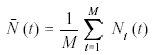(1)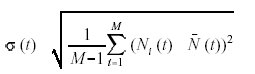(2)

(B.6)  Steps (B.1) - (B.5) are repeated for n = 2, ..., 181 corresponding to t = 0.5 to t = 90. As  with the real mound analysis (1d) two sets of precisions were used.

C. Discussion and Graphical Display of Results.

Our question is: How many of these right and associated isosceles triangles occur in a coordinated fit among these twelve mounds, and what is the relation of that number of occurrences to chance distribution? Although there are sixteen mounds in the area, as previously stated four of them are so close together in relation to their size that angular relations between them allow too much freedom for interpretation. We therefore restrict our procedure to twelve mounds, but take the four mounds into consideration later on.

To avoid possible bias toward any particular pair of right and isosceles triangles, or equivalently towards any particular angle  t, we have examined the set of all right and associated isosceles triangles by allowing t to vary in 1/2 intervals from 0 to 90. The graphical display of the results of our tests is in the form of a distribution and allows us to determine if there is any clustering of the number of such triangles about any particular value of t.

The results are displayed in graphs for two different levels of precision. For a five degree precision we plot (for the actual mounds) the number N(t) of coordinated fits of right and associated isosceles triangles having angles that agree with the ideal within less than 5. Then, for greater precision we plot  N(t) for triangles having angles that agree with the ideal within less than 0.2. For both degrees of precision we plot a comparison curve for the average number of triangles (t) that should occur by chance. This comparison curve is obtained by the simulations described above.

(C.1) First Plot: Five Degree Precision (Figure 9)

Figure 9 compares the plot for N(t) with the plot for (t) when the precision required for a coordinated fit among all twelve mounds is five degrees.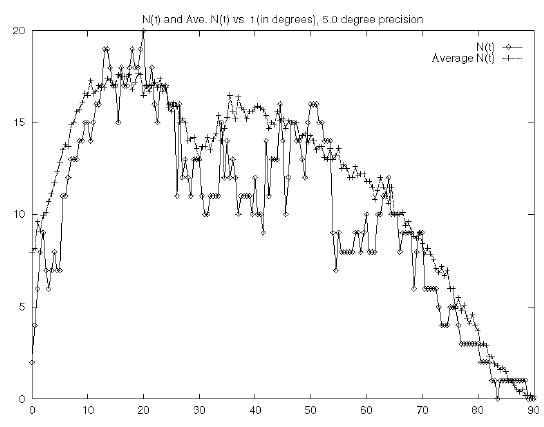Figure 1 N(t) and (t) versus t (in degrees), 5.0 degree precision
The  plot in Figure 9 for the actual number of triangles for each value of t shows a broad rise and tapering off from about 6E to about 30E. The plot for  N(t) in the same figure shows a similar rise and tapering off. For both curves we find a distinct dip or decrease in the number of triangles when t = 30. This dip reflects  the fact that the isosceles  triangles  become equilateral for t = 30 and triangles which are equilateral (or nearly so) are much less likely to occur by chance.

The two curves match reasonably well: At this level of precision there is no significant deviation from background and this result would tend to support the random geology hypothesis.  We remind the reader that the coordinated fit points are not restricted to the centers of the mounds but are allowed to vary within the perimeters of the mounds (subject however to the constraint of a coordinated fit). With this lower precision, that variation stops when the angles of the candidate triangles match those of either the ideal right triangle or ideal isosceles triangle within less than 5 degrees. When that coordinated fit variation continues until we achieve a higher precision, many candidate triangles fall by the wayside and if the Cydonia mound distribution was random it would likely fall along with the computer generated curve .

(C.2) Second Plot:  0.2 Degree Precision (Figure 10)

Instead, however, we find that when the precision is increased, a peak at t = 19.5 degrees emerges decisively from the background.  (This is close to our estimate of 19 degrees based on measurements of the initial   mounds ( see Figure 5)).  Figure 10 shows a well-defined peak of 19 occurrences at this angle (12 similar right and 7 similar isosceles triangles),  in the same region where a broad peak was seen in the lower precision plot.  In our twelve mound coordinated fit, the 6 mounds K,J,L,Q,M, and O to the north of G,A,B,D,E,P contribute 7 additional copies JPD, PGL, GKL, AEL, PLA, MQA, GKQ of the right  triangle similar to  EAG, DAG, ABD, EAB, and PGE seen earlier for 12 similar right triangles altogether.  In addition, those remaining mounds K,J,L,Q, M, and O contribute 5 additional copies KAE, PEL, QJG, KLQ, and GMO of the isosceles triangle similar to those of PMA and ADE described in Figures 3 and 8, yielding altogether 7 isosceles triangles with the same proportions.  Altogether  nineteen of these special right and isosceles triangles appear in our twelve mound coordinated fit (one vertex per mound restricted to the mound perimeter, not necessarily the center).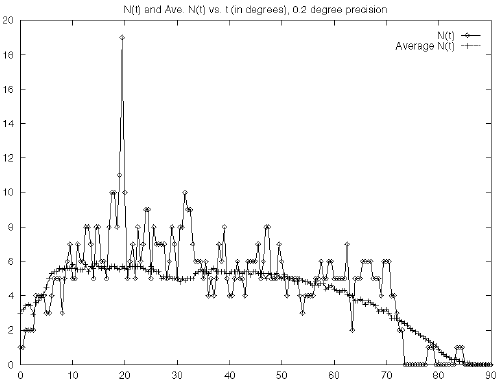Figure 10 N(t) and (t) versus t (in degrees), 0.2 degree precision

The comparison curve in the above plot  for the (t) random distribution retains the same general shape as it had for the lower precision, but as expected drops dramatically in scale, with its high plateau now reaching only as high as six occurrences as a result of the greater precision required. Any further increase in the precision to still smaller angle uncertainties would not change these plots significantly because of the finite mound size. The peak clearly indicates an anomaly. The actual mound distribution contains an inordinately high number (19) of  related right and isosceles triangles when t = 19.5. For a random distribution we would expect a graph more like Figure 9 where the signal is buried in the noise.  At the precision level of 0.2 degrees, we find that  =5.7 and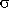=2.0).

4.  Level of Significance- an Anomaly of Number and Precision

Following up on the sharply defined differentiation between those triangles where t = 19.5 degrees and those for all other values of t, Figure 11 summarizes the results of 10,000,000 simulations of the random placements of 12 fictitious mounds among the larger features. The distribution function F(N) gives the number of times out of that total of 10,000,000 that there will be N appearances of the right and associated isosceles triangles for  t = 19.5 degrees. On the X axis we have the number N of such triangles and on the Y axis the number F(N) of random distributions of simulated mounds (computer runs) that produce that number N of triangles.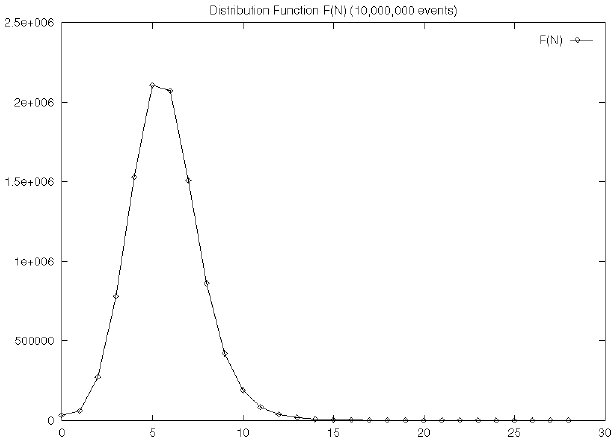Figure 11 The Distribution Function F(N) versus N
Looking  at the  curve  F(N) in Figure 11,  we see a curve centered about a mean value of  about 6 and width of about 2.  In contrast to these figures for random distributions, the value of N corresponding to the actual Cydonia data (19) occurs far to the right where the curve tapers off, effectively outside the curve, just under 7 standard deviations away from the mean.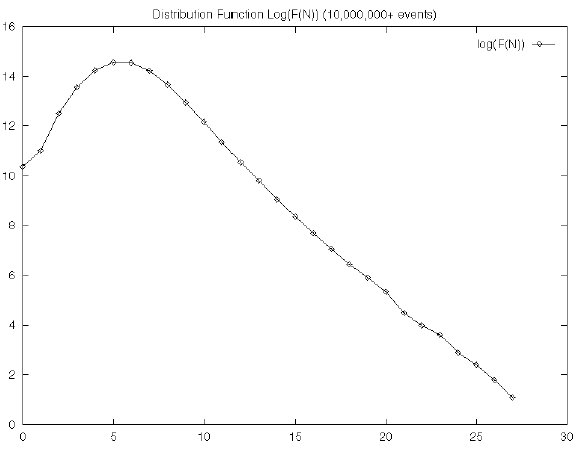Figure 12 The Distribution Function log(F(N) versus N

However, the distribution curve F(N) is not a normal curve.  This can be seen in the  plot of Figure 12 of log(F(N)) versus N.  If the distribution were normal then the log curve should be quadratic.  Instead for larger N we see a nearly linear curve. We speculate that the non-normal behavior occurs as N increases because  the constraints imposed by geometry on a finite number of mounds enhance appearances of still more of the ideal triangles.  Although we do not analyze the exact cause here, what this means is that we cannot estimate the probabilities of the actual frequency of 19 being the result of chance by extrapolating the  complimentary error function (associated with a normal curve) out to 7. Thus, we are forced to take a more direct, though very time consuming route.  We computed directly the odds or level of significance of our result.

In order to obtain a more representative  measure of the level of significance of our results we should take into account another important aspect or our coordinated fit, and that is the  precision with which  the coordinated fit points adhere to the center of the mounds. (The above two curves have no restriction on this measure). It was noticed early in our research that the more visibly obvious symmetries, such as those represented in Figure 3, have the further remarkable feature that the fit points are very close to the center of the mounds.  Like the number of redundant right and isosceles triangles this is also an anomaly relative to what one would expect, based on chance alone.  We found that the average distance     of the coordinated fit point from the measured centers of each of the 12 actual Cydonia mounds is less than the corresponding distances on the randomly generated data in almost 95% of the ten sets of one million computer simulations. This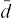is defined as the summation shown below.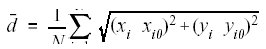(4)

in which here N is the number of mounds in the configuration (here there are 12 mounds);  xi0, yi0, are the coordinates of the estimated  center of the mound i; and xi,yi are the coordinated fit points within the mounds of the vertices of our desired triangles.

Thevalue  gives a quantitative measure of how close on average the coordinated fit point within each mound is to the center of that mound.  The smalleris, the closer the coordinates of vertices of the ideal triangles lie to the estimated  center of the  mound. A of 0.0 would indicate a fit with all vertices directly on the estimated mound center. Theneeded for a precise fit (within 0.2 degrees) of the model in the case of 19 triangles for the 12 mounds (in the case of t = 19.5)  was 3.45 pixels.  The larger majority of all computer simulated fits had> 3.45 pixels.

We need a measure of the level of significance that accounts for both anomalies simultaneously (that of number and precision).  We obtain that by modifying the definition of a  hit as defined as in item 2c) to include the requirement that thevalue for the coordinated fit to the fictitious mounds be less than or equal to thevalue for the coordinated fit to the actual  mounds. From our ten sets of 1 million simulations that we ran we found that on average, for one million simulations, the number of runs that gave 19 or more appearances of these right and isosceles triangles and  that had aless than or equal to 3.45 pixels (as in the case of the  actual mounds) was about 15.52.5. This represents a level of significance of about 0.0000155 and  is far less than the common choice of 0.01 used to reject the null hypothesis.  Based on this we state that the chances we are in error in rejecting the random geology (or null) hypothesis are extremely remote.

5.  Further Tests and Considerations

In this section we describe several additional tests that lend support to the anomalous nature of the mound distribution that we have found.

Earlier we noted that there are four mounds not taken into consideration, due to their being too tightly clustered in relation to their size, which would allow too great a degree of freedom in making angular measurement. These four mounds are arranged in a square or cross pattern, with a hint of another, fifth mound, at the center of this square. The area defined by the four mounds is approximately equal to the average size of the other mounds. Calling this area mound S, the analysis was run again for 13 mounds the original twelve and the area outlined by the four tightly clustered mounds. We found there is essentially no change in the level of significance.

B. Analysis of Unrelated Right and Isosceles Triangles

The analysis of triangle distributions presented above partners all right triangles (for t from 0 to 90 degrees) with a particular isosceles triangle. A question remains whether there are other favored patterns in the actual mound data involving other pairs of right and isosceles triangles, which unlike our above related right and isosceles, would have no particular relation,. Toward this end we have done coordinated fits to right and isosceles triangles defined by/2, (/4 + t/2), (/4 - t/2) and (/2 - t), (/4 + t/2), (/4 +t/2) with t varying from 0 to 90 degrees and  t varying independently from -90 to +90 degrees. Both variations are done in one-half degree steps. This includes combinations of  pairings of  all possible right and isosceles triangles including straight lines, (coordinated fits to three mounds lying in a straight line).

We find that the combination with the highest N(t,t) for the actual Cydonia mounds is again at  t=t=19.5 degrees. There are other pairings that are high (above 10) but in all cases they involve either t =19,19.5,20 and either  t =19,19.5,20, or t = -90,-89.5.  The latter two angles for the isosceles correspond  virtually to  straight lines. Thus, the most favored pairings of isosceles and right triangles, besides t=t=19.5 involve t=19.5, corresponding to right triangles with angles about 35.25,54.75,90 degrees, and degenerate isosceles triangles with angles of 0,0,180 degrees.

C. Analysis of Single Triangles with Arbitrary Angles

We next examine  the frequency of appearance of arbitrary triangles, including the large class of irregular triangles. Toward this end we have done coordinated fits on the actual mounds to triangles with angles t,u,-t-u.  We allow t to vary in one-quarter degree steps from 0 to 60 degrees and allow u to vary from t  to 90-t/2 or 90 -(t + 1)/2 also in one-quarter degree steps.  We find that there are two different triangles which most frequently appear (12 times).  One of them is the ubiquitous right triangles with  angles of/2, (/4 + t/2), (/4 - t/2) with  t =19.5 degrees, while the other is an obtuse triangle.  It is rather startling to find that the angles of that obtuse triangle are given in terms of the same value of  t, namely  t, (/4 - t/2), (3/4 - t/2) where again  t =19.5 degrees.  This tendency to favor geometry related to this particular value of  t points to an anomaly of geometry as well as one of number and precision.  This geometry will be examined more in a future paper.

Another test that could in principle be made would be to consider a fixed set of 1,000,000 randomly chosen 12-mound configurations and  one-by-one, make a comprehensive search for a striking repetitive or symmetric pattern on each. It could possibly be that a significant subset (i.e. significantly greater in number  than the 15.5 we found above) of such configurations would display  highly redundant (on the order of 19) patterns of some sort (though very unlikely to be the same pattern for all members of the subset  because the search would be comprehensive for each randomly chosen 12-mound configuration). This would not likely diminish the significance of our findings here.  The reason is that such a comprehensive study of the actual Cydonia mound configurations would  very likely show significantly more striking geometries, certainly  more repetitive than that of the 19 related right and isosceles triangles found in this paper. This is seen above where a redundant appearance of another (obtuse) , but  related triangle is found in the Cydonia configuration.  A detailed application of this test will be included of a future paper.

D.  An Anomaly of Location.

A further mathematical analysis outlined below indicates that the anomalies of number, geometry, and precision are compounded yet again. By  using  methods of analytic geometry it is possible to show that the configuration of the actual twelve mounds allows a coordinated fit of eleven prs and lrr triangles with p =/2 , r =/4 + t/2, s =/4 - t/2, l = 2s  with t in the restricted range from about 18.5 - 20.5 in one-half degree intervals with this number rising sharply to 19 for t = arcsin(1/3) =19.47.. .  At this precise value of the defining angle,  the grid location found in our geometrical analysis can be summarized briefly by the following table. It gives the X and Y coordinates of the coordinated fit points of the twelve mounds (and point S at the center of the four small mounds), transformed and uniformly scaled so that the coordinated fit point of mound G is at the origin and that of mound B at (X,Y) = (12,-12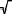2).

 G A D E B P K J L M O Q S 0,0 12,-12 6,-24 0,-12 12,-24 -12,0 4,4 2,4 0,12 6,18 -6,15 -2,4 0,6

This scale is chosen so that all of the coordinated fit points are of the form (Mi,Ni/2) with M and N integers presented in the table.  Applying  analytic geometry to the tabulated values of these fit points one can readily show that twelve three mound configurations are prs right triangles (EAG, GAD, ABD, EAB, PGE, JPD ,PGL ,GKL, AEL, PLA, MQA, GKO) while seven three mound configurations are lrr isosceles triangles (EAD, PMA, KAE, PEL, QJG, KLQ, GMO) with  t  = arcsin (1/3).  Figure 10 shows that  chance is unlikely to be responsible for the eleven appearances on either side of this angle much less  the sudden change in N to 19 at this value of the angle . Thus the actual mound placements must be anomalously configured in order for an already rare value of N = 11 to jump to N  = 19 for t = arcsin (1/3) . This sudden jump from 11 to 19 is a consequence not only of the special proportions of the right and isosceles triangles at that value of t but also the special locations of the actual Cydonia mounds. Without special locations or relative placements of the mounds the number of appearances would be closer to 11 at this angle. A detailed analysis of these claims will be included in a future paper, but to give an example of what we mean consider the effect of adding mound G to the three mound configuration corresponding to the isosceles triangle ADE.  Referring to Figure 3 we see that the addition of that one mound adds two right triangles (GEA and GAD). However, these three triangles correspond to two prs and one lrr triangle with a common value of t only for  t  = arcsin (1/3).  For other nearby values of t only one of the two right triangles can be partnered with the isosceles for the same value of t.  Here we see how the special proportions of the right and isosceles triangles leads to a jump in the number of appearances from 2 to 3. To see a simple example how a special location can lead to a jump consider the addition of a fifth mound to the four mound configuration GADE.  Mound P clearly would add just one prs right triangle.   Mound B would also clearly add a prs right triangle ABD. However, it also adds a second  prs right triangle ABE, but only for  t  = arcsin (1/3).  So as seen in this simple example, a special position is important in order to take advantage of  special proportions.

6. Conclusion:

In this paper we have established the existence of an anomaly on Mars in the form of angular placements of relatively small surface features we call mounds. The anomaly has four aspects: geometry, number, precision, and location. Beginning with the isosceles triad ADE of mounds and continuing through all twelve mounds in the vicinity we have redundant appearances of right and isosceles triangles. Compounding this anomaly is the finding that as we go from mound to moch as to take advantage of a peculiar feature of the geometry (the angle arcsin(1/3)) that defines the proportions of the triangles, increasing the number of appearances of these triangles in a coordinated fit.

We must conclude that the random geology hypothesis fails by a very large margin,  that a radical statistical anomaly exists in the distribution of mound formations in this area of Mars.Since  previous research in this area seemed to indicate possible anomalies (including, but not limited to the controversial Face), we had  reason to focus on this region. If we had chosen an area at random on Mars and found these mound relations then we should factor in  the area of the entire planet in our statistical calculations. But this would presuppose that on average all other regions of Mars had a similar density of mounds and that the only mound anomalies are at Cydonia. Our studies of numerous Viking  images shows that mounds of this type are far from ubiquitous.  The existence of this  radical statistical anomaly  in the distribution  of mound formations in this area of Mars indicates in our opinion a need for continued high priority targeting of the area for active investigation and determination of the origin and nature of the mounds.

BIBLIOGRAPHY

Brownlee, K.A., Statistical Theory and Methodology in Science and Engineering. John Wiley and Sons, Inc. New York, London, Sydney (1965)

Carlotto, Mark J., Digital Imagery Analysis of Unusual Martian Surface Features. Applied  Optics, Vol. 27, No. 10 (1988).

Carlotto, Mark J. And Stein, M. C., A Method for Searching for Artificial Objects on Planetary  Surfaces.  Journal of the British Interplanetary Society, Vol. 43 No. 5 (May 1990).

Carlotto, Mark J., Evidence in Support of the Hypothesis that Certain Objects on Mars are Artificial in Origin. J. Scientific Exploration,Vol. 11, No. 2, Summer 1997.

Carlotto, Mark J., The Martian Enigmas: A Closer Look. North Atlantic Books, Berkeley CA (1991, 1997).

Carr, Michael H., The Surface of Mars. Yale University Press, New Haven (1981).

Chandrasekhar S., Hydrodynamic and Hydromagnetic Stability.  Oxford Univ.  Press 1961 (Dover 1981) (Chapter 2 discusses the Bernard convection  problem).

Crater, Horace W., A Probabilistic Analysis of  Geomorphological Anomalies in the Cydonian Region of the Martian  Surface. Paper delivered in Cody, Wyoming at the  Moon/Mars conference (September 16, 1994)

Crater, Horace W., A Statistical Study of Angular Placements of Features on Mars. Paper delivered  at Society for Scientific Exploration meetings (June 15 1995).

Crater, Horace W., Anomalous Mound Distributions on the Cydonia Plain, Poster presentations delivered at the Spring meeting of American Geophysical Union, Boston (May 28,1998)

DiPietro, V., Molenaar, G., & Brandenburg, J., Unusual Mars Surface Features. Mars Research, PO  Box 284, Glenn Dale, MD 20769. (First edition 1982; fourth edition, 1988.)

DiPietro, V., Molenaar, G., & Brandenburg, J., The Cydonia Hypothesis.. Journal of Scientific  Exploration,  Vol. 5, No. 1, pages 1-25 (1991).

Erjavec, J., The Geomorphology and Geology of a Portion of the Cydonia Region of Mars: a New  Interpretation. Unpublished Manuscript.

Hoagland, Richard C., The Monuments of Mars: A City on the Edge of Forever. North Atlantic  Books, Berkeley, CA 94705. (1987, 1992.)

McDaniel, Stanley V., The McDaniel Report: On the Failure of Executive, Congressional, and Scientific Responsibility in Setting Mission Priorities for NASA's Mars Exploration Program. North  Atlantic Press, Berkeley CA (1994).

O'Leary, B., Analysis of Images of the  Face on Mars and Possible Intelligent Origin. Journal of the British Interplanetary Society, Vol. 43 No. 5 (May 1990).

Pittendrigh,Colin S; Vishniac,  Wolf  and Pearman, J. P. T. Editors; Biology and the Exploration of Mars, . Published by National Academy of Sciences National Research  Council, Washington, D C, 1966  The article "Remote detection of terrestrial life" is by Carl Sagan et al .  p.187 - 209.

Pozos, Randolfo R., The Face on Mars: Evidence for a Lost Civilization- Chicago Review Press,  814 N. Franklin, Chicago, IL 60610 (1986). Also available from North Atlantic Books, 2800 Woolsey Street, Berkeley, CA 94705.

Strange, James F. Some Statistical Observations on the Distance between some of the Mounds at Cydonia. Paper presented before the Society for Scientific Exploration at Charlottesville, VA, May 1996.

Stuart, J. T., On the Cellular Patterns in Thermal Convection   Journal of Fluid Mechanics, vol 18, pp 481-498, 1964.

Suppe, John, Principles of Structural Geology. Prentice  Hall, Edgerton, New Jersey (1985)

Torun, Erol, The Geomorphology and Geometry of the D & M Pyramid.  (1988). Appendices A and B added June and August 1989. Published electronically on the World Wide Web at the following address: http://www.well.com/user/etorun/pyramid.html.

van Dyke, Milton (Assembler), An Album of Fluid Motion. Parabolic  Press, Stanford CA (1982).

The authors state "attention was first drawn to these objects   because of the apparent regularity of arrangement... of the mounds P, G, E,   A, D and B.  They then carry out analyses of all mounds including P, G, E,   A, D and B.  This is not a valid procedure.  One should not use the same   data set to search for a pattern and to test for that pattern.  As Feynman   has remarked, the invalid procedure is like saying:  "I came here in a taxi   with the license plate LPG408.  This is quite remarkable.  What is the  chance, of all possible taxis in the city, I select by accident one with   license plate number LPG408?"  A valid procedure is to divide the data into   two parts and use one part to search for patterns, and the other part to   test for the significance of any pattern so found.

The same error appears to be repeated later on in relation to   Figure 11.  This gives the number of times that, out of 10 million trials,   one would get a particular number of occurrences of triangles with t = 19.5  degrees.  However, the figure 19.5 degrees came out of their study of the   mounds.  Here again, a more persuasive procedure would be to divide the   data set into two parts, one to search for the recurrent angle, and the   other to test to see if that value of the angle is statistically   significant.  Alternatively, one may test to see if it is surprising that   any angle can have the number of recurrences that are found in the actual data.

To many readers, a purely mathematical evaluation will not be all that   satisfying.  If it is possible, we would like to have exactly the same   procedure carried out for 100 other areas selected randomly or according to some well defined algorithm.  If this could all be done by computer,   without human intervention, so much the better.

The authors discussed how they selected the mounds.  It would be  better if the authors had recruited six or seven other scientists, provided  them with the photographs and the relevant criteria, asked them to  individually apply the criteria to the photographs, and then compared the   results.  Would they all have come up with the same number of mounds?  How  closely would they have agreed in their coordinates?

The Mars Global Surveyor is now observing Mars.  I understand that   the authors do not wish to wait until the new survey is completed to repeat   their analysis on MGS data.  However, it would be reassuring if they could   at least check the appearance and locations of whatever mounds appear in both surveys.

The authors use the term "random geology hypothesis."  This is an   unfortunate term to use.  Some geological formations are obviously very far   from random.  (Consider, for instance, the "Devil's Post Piles" in   Ireland.)  The authors are really testing whether the distribution is   random.  If they were to show convincingly that it is not, they may simply   have shown that this array of mounds is another geological formation that   is nonrandom.  Of course, that in itself could be interesting.

Horace W. Crater and Stanley V. McDaniel

We respond to each of the six objection paragraphs in turn.

OBJECTION 1:

Although the appearance of one special triangle  (with finite precision) would be no more significant than what  the cab customer observed, our sequence of six special triangles (with the analogy being of 6 nearby cab license numbers differing say by just the last digit) represents  an entirely different statistical category. So this Feynman analogy is simply not applicable here. More importantly however, we should emphasize that in the paper, we refer initially to six mounds PGEADB for pedagogical reasons, presenting as they do a particularly noticeable visual regularity. In fact our exploratory analysis did not begin or end with 6 but started with four and eventually included all 12 mounds.  What drew attention to the mounds initially were claims by another writer about an apparent isosceles and right triangle among just mounds EADG. When we examined that configuration we found an unexpected geometric peculiarity not noticed by the earlier writer: the existence of two similar right triangles (GEA and ADG) and one isosceles triangle (ADE) defined by just one angle. Predicting a priori upon this discovered peculiarity, it was subsequently confirmed that mounds B and P fall within the predicted pattern, and step by step as each further mound was taken into consideration, the predicted pattern was found to be evident among all twelve mounds. This legitimate sequence of discovery (see sections 2 and 3C.2 in the paper) notwithstanding, the sequential order or the mental process which one uses in analyzing the data has no bearing on the statistical significance of the pattern. What matters is whether or not there is any bias in the selection process and whether or not a pattern emerges that is statistically anomalous. We tested for all possible geometries of right and isosceles triangles.

OBJECTION 2:

The procedure followed to generate Fig. 10 is precisely what the referee has asked for in the latter part of his statement. Fig. 10 compares at each angle the number of recurrences found in the actual data with that based on a computer simulation. It thus tests to see if it is surprising that any angle can have the number of recurrences observed. It could have been that there were numerous geometries that showed surprising numbers of appearances as a result of generating Fig. 10, but what it clearly shows instead is that there is only one geometry, that determined by the angle 19.5 degrees, that stands out. Having found that we then did a computer analysis to determine just how unlikely that would have been. That was presented in Fig. 11.

OBJECTION 3:

It is incorrect to assert that our evaluation is ''purely mathematical'' (whatever that may mean). The redundancy  (isosceles and right triangles and numerous sets of parallel lines) shown  in Fig. 3 is visually compelling. Furthermore we surveyed about 60 other  similar sized regions on Mars and in none of them did we find these many similar sized mounds. When we did find images with a few, they displayed no compelling visual or measured symmetries as in Fig. 3. We also determined that about 4,000 triangle configurations defined by small craters from a nearby area displayed the same triangle recurrence frequency as our computer simulations based on random number generators. Thus having established that our method when applied to features that have a clear natural origin gives  the verdict random,  we finally examined millions of equivalent areas containing simulated mounds in millions of random distributions. This indeed was done ''by computer, without human intervention.''. The various tests for non-random distribution we have carried out stand on their own merit independently of what might be found elsewhere.

OBJECTION 4:

Appropriate criteria for mound selection was repeatedly discussed, critiqued, and refined over more than two years by members of the Society for Planetary SETI research. Other scientists studied the Viking images and helped establish the mound selection criteria. These criteria do not single out a biased subset, but all such objects in the area, within reasonable limits. We offer to supply independently orthorectified images to other scientists who wish to undertake replication of our work.

OBJECTION 5:

The last targeted pictures of Cydonia were taken in April 1998. Only two narrow strip images of the region were taken, and only four mounds appear in these images. Their relative locations are consistent with those displayed in the Viking data. No significant further study can be done until the MGS enters its mapping phase and returns more comprehensive coverage of the area.

OBJECTION 6:

The objection seems to assume that non-random geological formations are common, but this is not supported either qualitatively or quantitatively, nor is the method by which it would be determined stated. We are not aware of any studies, comparable to our own, carried out on terrestrial formations, that would identify such a degree of non-random distribution of separate objects. In discussions with geologists we have found none who could identify distributions of natural objects interrestrial terrain displaying such non-random characteristics. Our research poses the question "is there any known geological mechanism that could produce such coherent patterns among mound-sized objects over distances of kilometers?" Thus far no adequate geological answer has been forthcoming.Archaeologist J. F. Strange of the University of South Florida has stated on the basis of our data, and on the basis of his separately applied Kolgorov-Smirnov test, that if the mounds had been found on the Earth, archaeological teams would be strongly motivated to investigate them. We therefore believe our results are substantive and indicate that further serious study of the region should be undertaken.